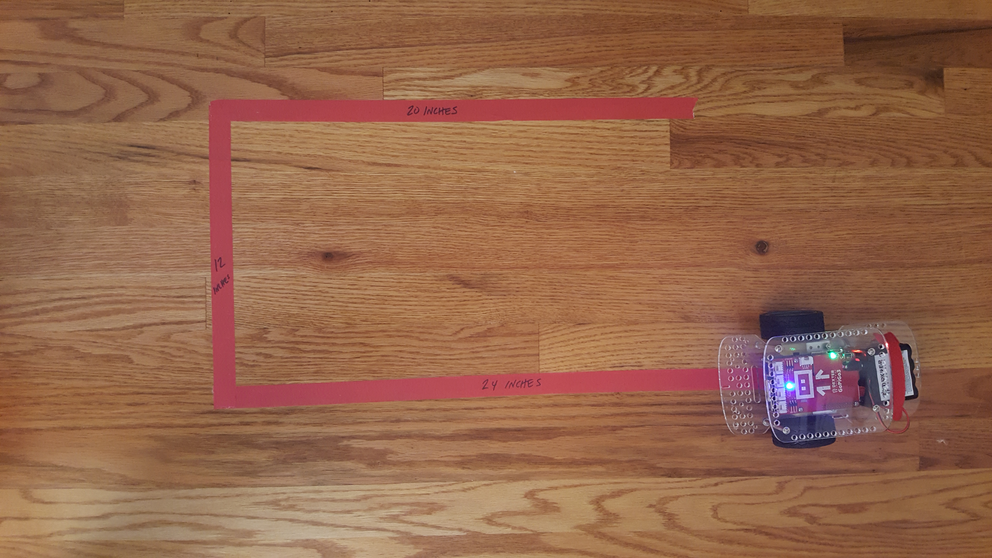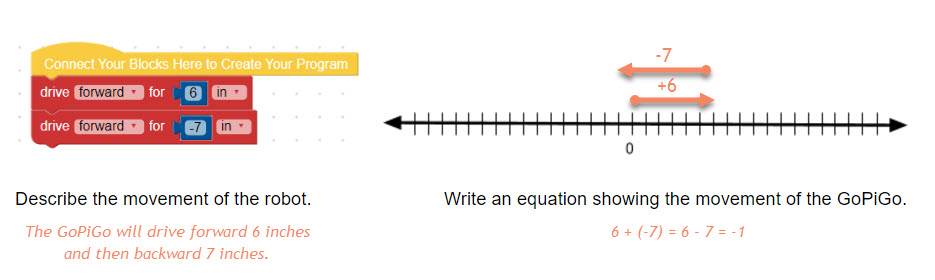## Adding and Subtracting Integers on a Number Line

Students will investigate adding and subtracting integers through programming the GoPiGo robot to move forward and backward on a number line.

Setup

1. Create number lines on the floor in inches. This can also be done by students during the lesson depending on the amount of time available.
2. Attach a straw, small slip of paper, or pipe cleaner to the front of each GoPiGo to serve as a marker on the number line. This can be attached to the front or slipped through one of the holes in the acrylic body of the GoPiGo (see image below).
3. Connect to one of the GoPiGo robots (the first two problems on the handout will be completed as a class)
4. Copy student handoutsIntroduction

1. Ask students, "What is an integer?"
2. Tell students that the word integer comes from Latin meaning 'whole' and 'entire'. An integer is defined as "any of the natural numbers, the negatives of these numbers, or zero" (Merriam-Webster).
3. Ask the students, "What does that mean? What are some examples of integers?"
4. Tell the students, "Today we are going to learn about how to add and subtract positive and negative integers."

Procedure

1. Ask the class, "What does addition mean?" (ex. put together, more, combine) "What does subtraction mean?'" (ex. take away, remove)
2. Tell the class, we are going to be programming robots to move along number lines today to illustrate addition and subtraction of integers. Ask, which operation will be represented by the drive forward block? (Addition) Which operation will be represented by the drive backward block? (subtraction)
3. Complete the first problem or two as a class. Connect to a GoPiGo robot and then go to 'Code in Bloxter'. Duplicate the first program on the student handout. Place the robot on the number line with the marker on 0 and the front of the robot (the side without the battery pack) facing the positive side of the number line.
4. Ask the students, "What do you expect the robot to do?"
5. Run the program and discuss what the robot did, illustrate the movement on the number line, and write an equation showing the movement of the GoPiGo as well as the final position of the robot.
6. Students will then work in small groups to complete the same process for the rest of the problems on the student handout.
7. After completing the problems with premade programs, they will write their own programs and then again describe the movement in words, on a number line, and using an equation.
8. Students will analyze their work to determine rules for adding and subtracting integers. Discuss student observations and ensure students have the correct procedures for adding and subtracting integers.Closure

1. Facilitate a class discussion where students reflect on the process. What did you learn? What was easy to understand? What was difficult to understand? What were challenges? What surprised you? What questions do you still have?